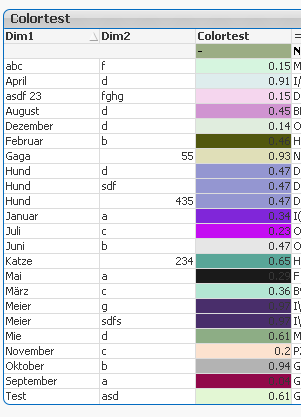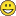# QlikView App Dev

Discussion Board for collaboration related to QlikView App Development.

Announcements
Join this live chat April 6, 10AM EST - QlikView to Qlik Sense REGISTER
cancel
Showing results for
Did you mean:Contributor III

## Convert strings into RGB

Hi all,

Need some help on a tricky problemBasically we have a bar chart which is dynamically when it comes to the dimension:

DimensionFilterTable:

TableSelection, DimDisplayName

dim1, Country

dim2, Ciy

dim3, Seller

];

In the table we're working with a calculated dimension:

=\$(=TableSelection)

So far it works perfect. But now I want to use different colors for the different dimension elements - the normal approach doing this in the loading script is not really useful because this DimensionFilterTable is flexible. My idea was now to somehow generate RGB depending on the string:

 Element Color Exa1 red() Exb2 green() sdf black() Exa1 red()

Any idea? No script code should be used because it would mean to specify all the times the flexible dimensions and its elements with color codes. Something like this...but qlikishHash string into RGB color - Stack Overflow

1 Solution

Accepted SolutionsMVP

Uhm, wow. I hope you didn't spend much time on putting that monster together.

Perhaps this will work too:

hsl(

FieldIndex('\$(TableSelection)',[\$(TableSelection)])/FieldValueCount('\$(TableSelection)'),

0.5,

0.5

)

But imho a better idea is to load a table with colors like this one: List of Crayola crayon colors - Wikipedia‌. You could use the index value of the dimension to pick the color from the color table with the same index value.

talk is cheap, supply exceeds demand
7 RepliesMVP

Which color should be assigned to which dimension value? Is there a logic or do you simply want a random color assigned, any color will do as long as it is a color and each dimension value gets its own unique color?

talk is cheap, supply exceeds demandMVP & Luminary

Maybe this is helpful: Colors in charts.

- MarcusMVP

" the normal approach doing this in the loading script is not really useful because this DimensionFilterTable is flexible"

if this is your primary concern, you should be able to direct QV to the correct color code field (e,g. following Henric's blog post Marcus referenced) based on the dimension used with a similar approach you are using for the dimension:

DimensionFilterTable:

TableSelection, DimDisplayName , DimColorCodeField

dim1, Country, CountryColor

dim2, Ciy, CityColor

dim3, Seller, SellerColor

];

=\$(=Only(DimColorCodeField))Contributor III
Author

Exactly - at the end we're displaying always the same points on a map but the different classifications of the elements should be seenable with a different color. The classification is in different dimensions...

Guess I found an easy solution:

hsl(

(

mod((ord(mid(Hash128(Dim1),3,1)) + (((ord(mid(Hash128(Dim1),2,1)) + (((ord(mid(trim(Hash128(Dim1)),len(trim(Hash128(Dim1)))-1,1)) + ((0 << 5) - 0)) << 5) - (ord(mid(trim(Hash128(Dim1)),len(trim(Hash128(Dim1)))-1,1)) + ((0 << 5) - 0)))) << 5) - (ord(mid(Hash128(Dim1),2,1)) + (((ord(mid(trim(Hash128(Dim1)),len(trim(Hash128(Dim1)))-1,1)) + ((0 << 5) - 0)) << 5) - (ord(mid(trim(Hash128(Dim1)),len(trim(Hash128(Dim1)))-1,1)) + ((0 << 5) - 0)))))),100)

) /100

,

mod(ord(mid(Hash128(Dim1),5,1)),10)/10,mod(ord(mid(Hash128(Dim1),8,1)),10)/10)

Where Dim1 is replacable by the \$(TableSelection).

@Marcus / Stefan: This means I have:

a) always the same color for the different elements

or

b) I need to create a color mapping first with looping trough the elementsContributor III
Author

Result:MVP

Uhm, wow. I hope you didn't spend much time on putting that monster together.

Perhaps this will work too:

hsl(

FieldIndex('\$(TableSelection)',[\$(TableSelection)])/FieldValueCount('\$(TableSelection)'),

0.5,

0.5

)

But imho a better idea is to load a table with colors like this one: List of Crayola crayon colors - Wikipedia‌. You could use the index value of the dimension to pick the color from the color table with the same index value.

talk is cheap, supply exceeds demandContributor III
Author

Ok wow - that's a lot smarterThanks, works like a charm.